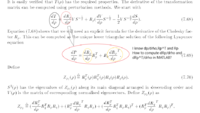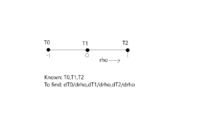# Finding derivative of Matrix at different grid points using Finite difference methods/ Cholesky Factorization

#### Sandeep Parameshwara

##### New member
It would be a huge help if someone can suggest to me how I can approach this problem. I need to solve the below-highlighted equation and then code it in MATLAB.
I need 'dT/drho'. For that, I need to solve for 'dRp/drho'. I do have a dependence on 'rho' but I don't have it explicitly. I have grid points, where I know the values of 'T, P, dP/drho, Rp, Rp^T, Rq and Rq^T' (which are all matrices at those grid points). I also know analytical 'P' (P=P0+P1*rho). How can I find 'dRp/drho'? I do think of finite difference methods. However, I can't calculate 'dT/drho' at the endpoints of the grid, I have to leave either starting or endpoint on the grid(by considering backward difference or forward difference method) Do I have to extend my grid in that case? or is it possible to code this in MATLAB using 'Cholesky factorization' or something? Because the text below mentions the "unique lower triangular solution" which made me think about Cholesky factorization. So, as I understand there are two ways to solve this problem.
1. Analytic solution using the below equation.
2. Finite difference method to find dT/drho avoiding all these equations.

I would like to understand from you whether it's possible to code these analytical equations. If not, how can I effectively use finite difference methods? Please feel free to ask questions if you are unclear about the question.Thank you!
sandeep